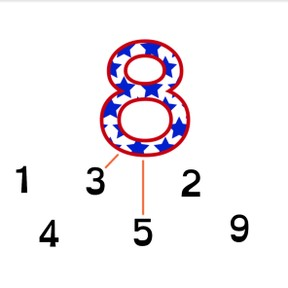8,000 schools use Gynzy92,000 teachers use Gynzy1,600,000 students use Gynzy

## General

The students can determine which addends, when taken together add to numbers up to 10.

## Relevance

If students want to be able to calculate well, they need to learn which numbers together add up to 10. It helps them to improve their addition and subtraction skills. You can make a group of 5 kids with 4 boys and 1 girl, but also with 3 boys and 2 girls, and also with 5 boys and 0 girls.

## Introduction

Show the students the image on the interactive whiteboard where they can count objects. Then have them count dots on the ladybugs. Ask students to count out loud.

## Development

Discuss with students that you can compose numbers from different amounts. Show this by counting the dots on the ladybugs. All sets of ladybugs have 5 dots. Use these to explain that a number can be made up of more than two smaller numbers. Show the different ladybugs and ask which ladybugs have 4 dots together. First search through the pairs of ladybugs to find 4 dots, then look through the trios of ladybugs. You can erase the grey boxes to check your answers.
Next, you will divide numbers into smaller groups by dragging dots onto the ladybugs. First divide the number into two, and then in the same way into three smaller numbers.
Next, explain how students can determine which smaller numbers make up a larger number, as shown by the shoes on the interactive whiteboard. The students first count the first number from the number bank, and count how many are left. If that number is written in the number bank, they can select it, but if not, they must try again with another number. Discuss that numbers can be made up of more than two numbers, as shown by the example with tubes. The students count using one of the given numbers and determine which numbers can be grouped to add to the total number of tubes.
As a final exercise, students must determine which two numbers add to 8, and which three numbers can be added to the total of 6, without visual support. Students who still need support with this can use blocks or other manipulatives.

Check that students understand grouping numbers to 10 by asking the following questions:
- What do you do if a number is made up of more than two numbers?
- Which numbers can 5 be made up of?
- Can 8 be made of 3 numbers? Which ones?

## Guided practice

Students first practice adding to a number with two addends with visual support. Students then practice coming up with alternate compositions of numbers, and finally must determine groups of three numbers to add to a given total.

## Closing

Repeat the learning goal and ask students what to do if a number is made of more than two smaller numbers. You can also ask students which numbers together make 5, and if you can find 3 numbers that add to 8. Play a game with the class. Divide the class into groups of 3 or 4. Give each group a set of number cards to 10. A student turns over a number card, and the first student who can name two numbers that add to total that number wins the card. The student with the most cards at the end wins. You can also play the game to require students to name three numbers that add to total the shown number.

## Teaching tips

Students who have difficulty with adding can be supported by using manipulatives like blocks. Have them practice by setting down the total in blocks, and then moving the blocks to form groups without adding or removing any other blocks.

## Instruction materials

Number cards to 10, blocks

### The online teaching platform for interactive whiteboards and displays in schools

• Save time building lessons

• Manage the classroom more efficiently

• Increase student engagement